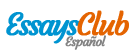# Entrevista Ing. Rodrigo Regalado EVAP.

Enviado por   •  27 de Diciembre de 2017  •  3.083 Palabras (13 Páginas)  •  80 Visitas

Página 1 de 13

...

The graph of each of these rational functions possesses a vertical asymptote. But bear in mind that the solutions of the IVPs

[pic 51]

Are defined on special intervals. They are respectively,

[pic 52]

The solution curves are portions of the graphs in b) and c) shown in blue. As predicted by the phase portrait, for the solution curve as ; for the solution curve in c), as from the left.” Cited directly from [pic 53][pic 54][pic 55][pic 56]

Attractors and repellers 

Suppose that y(x) is a non-constant solution of the autonomous differential equation dy/dx=f(x,y) and that C is a critical point of the differential equation. There are 3 types of behavior that y(x) can exhibit near our critical point:

- When both arrowheads on either side of the dot labeled c point toward c, all solutions y(x) of dy/dx=f(x,y) that start from an initial point (x0,y0) sufficiently near c exhibit asymptotic behavior when . For this reason the critical point c is said to be asymptotically stable. In this case c is also referred as an attractor.[pic 58][pic 57]

- When both arrow heads on either side of the dot c point away c, all solutions y(x) of dy/dx=f(x,y) that start from (x0,y0) move away from c as x increases. In this case the critical point c is said to be unstable. An unstable critical point is also called repeller.

- & d) The critical point illustrated on these cases neither an attractor nor a repeller. But since c exhibits characteristics of both, it is said that this point is semi-stable

Autonomous DEs and Direction Fields 

If a first-order differential equation is autonomous, then we see from the right-hand side of its normal form that slopes of lineal elements that pass through points in the rectangular grid used to construct a direction field for the DE depend solely on the y-coordinate of the points. In other words, lineal elements passing through points on any horizontal line must all have the same slope; slopes of lineal elements along any vertical line will, of course, vary. For example:[pic 60][pic 59]

Stable Critical Points

Let X1 be a critical point of an autonomous system and X=X(t), the solution that satisfies X(0)=X0; where . It is said that X1 is a stable critical point when, for any given radius , there is a corresponding radius r>0 such that if the initial position X0 satisfies , the corresponding X(t) solution satisfies for every t>0. If it also satisfies that and that in all cases , it is said that X1 is an asymptotic sable critical point.[pic 67][pic 61][pic 62][pic 63][pic 64][pic 65][pic 66]

NB. To emphasize that X0 must be selected close to X1, the terminology locally stable critical point is also used

Unstable Critical Points

Being X1 a critical point of an autonomous system and X=X(t), the solution that satisfies X(0)=X0; where . It is said that X1 is unstable if a disc of radius with radius , with the property that for every r>0 there is, at least, one initial position that satisfies , but the corresponding solution for at least one t>0.[pic 72][pic 68][pic 69][pic 70][pic 71]

If a point X1 is unstable, no matter how small is the neighborhood about X1, it will always find an initial position X0 that results in in a disc of radius on some time t on the future. Therefore unstable nodes, unstable spiral points and saddle points are examples of unstable critical points for linear systems. [pic 73]

For example:

Show that (0,0) is a stable critical point of the flat, non-linear, autonomous system

[pic 74]

The solution of the system on polar coordinates is:

[pic 75]

And if [pic 76]

Observe that and r tends to (0,0) as t grows. Therefore, given that , the solutions starts from a point units from the point (0,0) and it stays inside for . The point (0,0) is not only stable, it is asymptotically stable as it approaches to the point (0,0) as t tends to infinity. [pic 77][pic 78][pic 79][pic 80][pic 81]

Linearization

A linearization of a differentiable function f at a point is the equation of the tangent line to the graph of f at the point:[pic 82]

[pic 83]

For all the x values close to x1 on the graph of f are going to be points near the tangent line so the values of y(x) obtained by the tangent line equation are local linear approximations, in other words approximate the values of the function f(x).

It is unusual to determine the stability of a critical point of a nonlinear system by determining explicit solutions. Instead, the term g(x) is replaced on the original autonomous system X’=g(X) for a linear for a linear term A(X-X1) which is as close as possible from g(x) on the neighborhood X1.

A straight tangent line to the curve y=g(x) on X=X1 is y=g(X1)+g’(X- X1) if X1 is a critical point of X’=g(x) we have[pic 84]

Problems

- In a mathematical model for the control of ovulation, Lacker (1981) proposes the equation in this equation x represents the amount of hormones and is a constant that can be either positive or negative.[pic 85][pic 86]

- Sketch as a function of x:[pic 87]

[pic 88]

(Graph 1.1 for )[pic 89][pic 90]

[pic 91]

(Graph 1.2 for )[pic 92][pic 93]

[pic 94]

(Graph 1.3 for )[pic 95][pic 96]

- Analytically compute the location of all fixed points

- for ; ; ;[pic 97][pic 98][pic 99][pic 100]

[pic 101]

;[pic 102]

; [pic 103][pic 104]

- for ; ; ; [pic 105][pic 106][pic 107][pic 108][pic 109]

...

Descargar como  txt (18.6 Kb)   pdf (72.4 Kb)   docx (23.8 Kb)
Leer 12 páginas más »
Disponible sólo en Essays.club